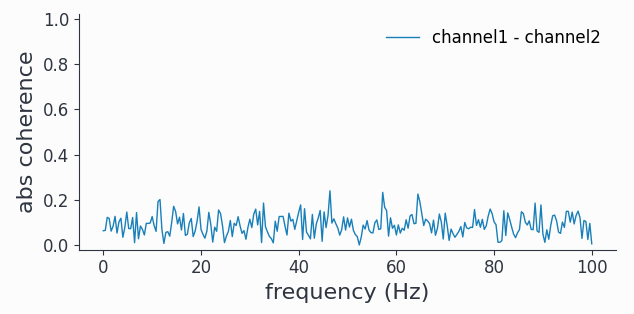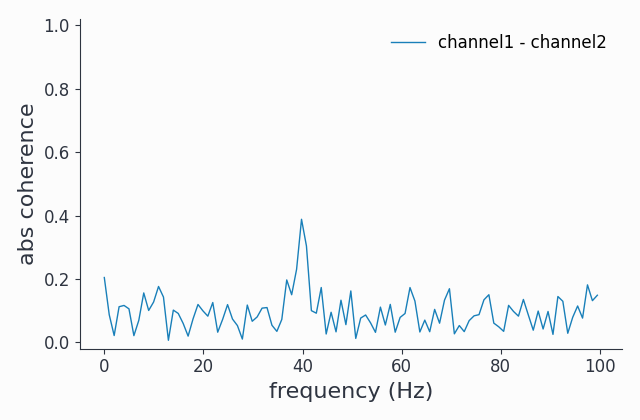# Selections#

Basically every practical data analysis project involves working on subsets of the data. Syncopy offers the powerful `selectdata()` function to achieve exactly that. There are two distinct ways to apply a selection, either in-place or the chosen subset gets copied and returned as a new Syncopy data object. Additionally, every Syncopy meta-function (see Syncopy Meta-Functions) supports the `select` keyword, which applies an in place selection on the fly when processing data.

Selections are an important concept of Syncopy and are being re-used under the hood for plotting functions like `singlepanelplot()` and also for `show()`. Both plotting and numpy array extraction naturally operate on subsets of the data, and conveniently use the same selection criteria syntax as `selectdata()`.

## Creating Selections#

Syncopy data objects can be best understood as n-dimensional arrays or matrices, with each dimension holding a certain property of the data. For an `AnalogData` object these dimensions would be `time`, `channel` and `trials`. Now we can define sub-slices of the data by combining index sets for each of those axes.

A new selection can be created by calling `selectdata()`, here we want to select a single trial and two channels:

```trial10 = AData.selectdata(trials=10, channel=["channel11", "channel17"])
# alternatively
trial10 = spy.selectdata(AData, trials=10, channel=["channel02", "channel06"])
```

`AData` is an `AnalogData` object, and hence the resulting `trial10` data object will also be of that same data type. Inspecting the original dataset by simply typing its name into the Python interpreter:

```AData
```

we see that we have 100 trials and 10 channels:

```Syncopy AnalogData object with fields

cfg : dictionary with keys ''
channel :  element <class 'numpy.ndarray'>
container : None
data : 100 trials of length 500.0 defined on [50000 x 10] float32 Dataset of size 1.91 MB
...
```

If we now inspect our selection results:

```trial10
```

we see that we are left with 1 trial and 2 channels:

```Syncopy AnalogData object with fields

cfg : dictionary with keys ''
channel :  element <class 'numpy.ndarray'>
container : None
data : 1 trials of length 500.0 defined on [500 x 2] float32 Dataset of size 0.00 MB
...
```

As we did not specify any selection criteria for the time axis (via `latency`) every sample was selected. This is true in general: whenever a certain dimension has no selection specification the complete axis is selected.

Finally by inspecting the `.log` (see also Trace Your Steps: Data Logs) we can see the selection settings used to create this dataset:

```trials10.log
```
```write_log: computed _selectdata with settings
inplace = False
clear = False
latency=None
trials = 10
channel = ['channel02', 'channel06']
```

This log is persistent, meaning that when saving and later loading this reduced dataset the settings used for this selection can still be recovered. The table below summarizes all possible selection parameters and their availability for each datatype.

## Table of Selection Parameters#

There are various selection parameters available, which each can accept a variety of Python datatypes like `int`, `str` or `list`. Some selection parameters are only available for data types which have the corresponding dimension, like `frequency` for `SpectralData` and `CrossSpectralData`.

Parameter

Description

Accepted Types

Examples

Availability

trials

trial selection

int, array, list

`selectdata(trials=7)`

`selectdata(trials=[2, 9, 21])`

`selectdata(trials=np.arange(2, 10))`

all data types

channel

channel selection

int, str, list, array

`selectdata(channel=7)`

`selectdata(channel=[11, 16])`

`selectdata(channel=np.arange(2, 10))`

`selectdata(channel=["V1-11, "V2-12"])`

`AnalogData`

`SpectralData`

`CrossSpectralData`

`SpikeData`

latency

time interval of interest in seconds

list, float, ‘maxperiod’, ‘minperiod’, ‘prestim’, ‘poststim’

`selectdata(latency=[0.2, 1.])`

`selectdata(latency=0.5)`

`selectdata(latency='minperiod')`

`AnalogData`

`SpectralData`

`CrossSpectralData`

`SpikeData`

frequency

frequencies of interest in Hz

float, list

`selectdata(frequency=20.5)`

`selectdata(frequency=[5, 10, 15])`

`SpectralData`

`CrossSpectralData`

unit

unit selection

int, str, list, array

`selectdata(unit=7)`

`selectdata(unit=[11, 16, 32])`

`selectdata(unit=["unit17", "unit3"])`

`SpikeData`

eventid

eventid selection

int, list, array

`selectdata(eventid=2)`

`selectdata(eventid=[2, 0, 1])`

`EventData`

Note

Have a look at Data Basics for further details about Syncopy’s data classes and interfaces

## Inplace Selections#

An in-place selection can be understood as a mask being put onto the data. Meaning that the selected subset of the data is actually not copied on disc, but the selection criteria are applied in place to be used in a processing step. Inplace selections take two forms: either explicit via the `inplace` keyword `selectdata(..., inplace=True)`, or implicit by passing a `select` keyword to a Syncopy meta-function.

To illustrate this mechanic, let’s create a simulated dataset with `phase_diffusion()` and compute the coherence for the full dataset:

```import numpy as np
import syncopy as spy
from syncopy.tests import synth_data

# 100 trials of two phase diffusing signals with 40Hz
freq=40,
samplerate=200,
nSamples=500,
nChannels=2,
eps=0.01)

# coherence for full dataset

# plot coherence of channel1 vs channel2
coh1.singlepanelplot(channel_i='channel1', channel_j='channel2')
```Phase diffusing signals decorrelate over time, hence if we wait long enough we can’t see any coherence.

Note

As an exercise you could use `freqanalysis()` to confirm that there is indeed strong oscillatory activity in the 40Hz band

### Explicit inplace Selection#

To see if maybe for a shorter time period in the beginning of “the recording” the signals were actually more phase locked, we can use an in-place latency selection:

```# note there is no return value here
```

Inspecting the dataset:

```Syncopy AnalogData object with fields

cfg : dictionary with keys 'selectdata'
channel :  element <class 'numpy.ndarray'>
container : None
data : 100 trials defined on [50000 x 3] float64 Dataset of size 1.14 MB
dimord : time by channel
filename : /home/whir/.spy/spy_3e83_a9c8b544.analog
info : dictionary with keys ''
mode : r+
sampleinfo : [100 x 2] element <class 'numpy.ndarray'>
samplerate : 200.0
selection : Syncopy AnalogData selector with all channels, 201 times, 100 trials
tag : None
time : 100 element list
trialinfo : [100 x 0] element <class 'numpy.ndarray'>
trialintervals : [100 x 2] element <class 'numpy.ndarray'>
trials : 100 element iterable
```

we can see that now the `selection` entry is filled with information, telling us we selected 201 time points.

With that selection being active, let’s repeat the connectivity analysis:

```# coherence with active in-place selection

# plot coherence of channel1 vs channel2
coh2.singlepanelplot(channel_i='channel1', channel_j='channel2')
```Indeed, we now see some coherence around the 40Hz band.

Finally, let’s wipe the inplace selection before continuing:

```# inspect active inplace selection
>>> Syncopy AnalogData selector with all channels, 201 times, 100 trials

# wipe selection
```

### Inplace selection via `select` keyword#

Alternatively, we can also give a dictionary of selection parameters directly to every Syncopy meta-function. These will then internally apply an inplace selection before performing the analysis:

```# coherence only for selected time points
coh3 = spy.connectivityanalysis(adata, method='coh', select={'latency': [-1, 0]})

# plot coherence of channel1 vs channel2
coh3.singlepanelplot(channel_i='channel1', channel_j='channel2')
```Hopefully not surprisingly we get to exactly the same result as with an explicit in-place selection above. The difference here however is, that after the analysis is done, there is no active in-place selection present:

```adata.selection is None
>>> True
```

Hence, it’s important to note that implicit selections get wiped automatically after an analysis.

In the end it is up to the user to decide which way of applying selections is most practical in their situation.

## Relation to `show()` and `singlepanelplot()`#

As hinted on in the beginning of this chapter, both plotting and numpy array extraction adhere to the same syntax as `selectdata()`. Meaning that the following two arrays hold the same data:

```# First explicitly select a subset
trial10 = spy.selectdata(AData, trials=10, channel=["channel02", "channel06"])
# show everything of the subset
# WARNING: don't do this with large datasets!
arr1 = trial10.show()

# calling show() with the same selection
# criteria directly on the original complete dataset

# this is True!
arr1 == arr2
```

And in the same spirit, both plotting commands below will produce the same figure:

```# First explicitly select a subset
trial10 = spy.selectdata(AData, trials=10, channel=["channel02", "channel06"])
# plot everything: only 1 trial and 2 channels left
trial10.singlepanelplot()

# directly plot from full data set with same selection criteria